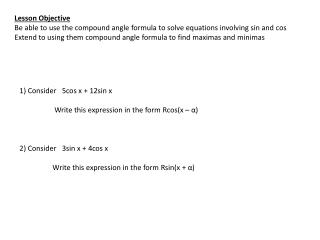DownloadDownload PresentationLesson Objective

# Lesson Objective

Télécharger la présentation## Lesson Objective

- - - - - - - - - - - - - - - - - - - - - - - - - - - E N D - - - - - - - - - - - - - - - - - - - - - - - - - - -
##### Presentation Transcript

1. Lesson Objective Be able to use the compound angle formula to solve equations involving sin and cos Extend to using them compound angle formula to find maximas and minimas 1) Consider 5cos x + 12sin x Write this expression in the form Rcos(x – α) 2) Consider 3sin x + 4cos x Write this expression in the form Rsin(x + α)

2. 1) 2) 3) 4) 5)

3. 2)

4. 2) 5)